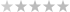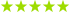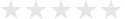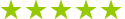# Calculus lessons in Switzerland

## 15 calculus teachers in Switzerland

0 teachers in my wish list

## 15 calculus teachers in Switzerland(2 reviews)
Danilson - Aigle29Fr
Math · Calculus · Science(3 reviews)
Eli - Zurich27Fr
Math · Algebra · Calculus(1 review)
Marina - Lausanne81Fr
Calculus · Math · Business accounting(3 reviews)
Calculus · Math(2 reviews)
Lisjana - Zürich (Kreis 3)33Fr
Trusted teacher: I specialize in tutoting calculus to international school students as well as national schools in Switzerland and Italy. My teaching method is a combination of theory and practice. The students can bring their own exercises to solve or to better understand. I provide students with extra exercises that can be done in class or as homework. The subject of the classes is the entire AP calculus program: 1) Limits and continuity: - Graphing and interpreting graphs (pre-calculus). - Limits and continuity. Finding limits algebraically or estimating them from numerical or graphical data. Continuity in terms of limits. - Intermediate Value Theorem and Extreme Value Theorem. - Vertical, horizontal, and oblique asymptotes. Limits involving infinity. 2) Derivates and rates of change: - Limit definition of the derivative and its relationship to continuity. - Derivative rules including the Power Rule, Product Rule, Quotient Rule, and Chain Rule. - Slope and tangent lines. - Linear approximation and differentials. - Instantaneous and average rates of change. Relationship among position, velocity, and acceleration functions. - Higher order derivatives. - Implicit Differentiation. - Analysis of Graphs based on both pre-calculus methods and derivative information. This includes finding intervals of increase/decrease, relative minima/maxima, intervals of concavity, and inflection points. - Mean value theorem and Rolle’s Theorem. - Applications of derivatives, including optimization and related rates. - Elementary differential equations and slope fields. 3) Integrals and Areas: - Antidifferentiation and indefinite integrals. - Techniques of antidifferentiation, including power rule, algebraic manipulation, and substitution. - Finite Riemann sums and their limits. Relationship to definite integrals. - The Fundamental Theorem of Calculus and definite integrals. - Trapezoid Rule and other methods for estimating area. - Exact area below a curve or between two curves, using definite integrals. - Volumes of solids of revolution, by washer method and shell method. - Accumulation functions. - Relationships between position, velocity, and acceleration using integrals. - Average value of a function over an interval. - Models for exponential growth and decay.
Calculus
Showing results 1 - 15 of 151 - 15 of 15

## Our students from Switzerland evaluate their Calculus teacher.

### To ensure the quality of our Calculus teachers, we ask our students from Switzerland to review them.Only reviews of students are published and they are guaranteed by Apprentus.Rated 4.9 out of 5 based on 11 reviews.

Statistics, Econometrics (including calibration of models on real data) (Adliswil)
GianmarcoGianmarco helped me understand and solve my problems in a friendly, quick and clear manner. He displayed a thorough understanding of the topic. I very much appreciate him as a teacher and can recommend him to anyone.
Review by CHRISTINA
Math tutoring in English. Let's make math fun and easy! (Helsinki)
EliEli is a thoughtful and experienced teacher. She is very creative in her approach, engaging from the very beginning of the lesson. My daughter is very happy and wants to keep learning with her.
Review by EVA
Mathematics, Statistik, Economics for both high school and university (Zürich (Kreis 3))
LisjanaWe are very happy with the help Lisjana is giving the girls, thank you
Review by GEORGINA